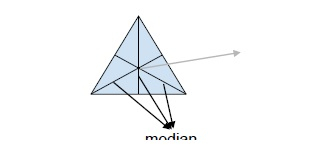# Program to find the Centroid of the Triangle in C++

C++Server Side ProgrammingProgramming

In this problem, we are given a 2D array that denotes coordinates of three vertices of the triangle. Our task is to create a program to find the Centroid of the Triangle in C++.

Centroid of a triangle is the point at which the three medians of the triangles intersect.

Median of a triangle is the line that connects the vertex of the triangle with the center point of the line opposite to it.Let’s take an example to understand the problem,

## Input

(-3, 1), (1.5, 0), (-3, -4)

## Output

(-3.5, -1)

## Explanation

Centroid (x, y) = ((-3+2.5-3)/3, (1 + 0 - 4)/3) = (-3.5, -1)

## Solution Approach

For solving the problem, we will use the geometrical formula for the centroid of the triangle.

For points (ax, ay), (bx, by), (cx, cy)

Centroid, x = (ax + bx + cx) / 3
y = (ay + by + cy) / 3

Program to illustrate the working of our solution,

## Example

Live Demo

#include <iostream>
using namespace std;
int main() {
float tri = {{-3, 1},{1.5, 0},{-3, -4}};
cout<<"Centroid of triangle is (";
cout<<((tri+tri+tri)/3)<<" , ";
cout<<((tri+tri+tri)/3)<<")";
return 0;
}

## Output

Centroid of triangle is (-1.5 , -1)